RRB JE CBT 1 - Full Test 7

# RRB JE CBT 1 - Full Test 7

Test Description

## 100 Questions MCQ Test RRB JE (Railways Junior Engineer) CBT Mock Test Series | RRB JE CBT 1 - Full Test 7

RRB JE CBT 1 - Full Test 7 for Railways 2023 is part of RRB JE (Railways Junior Engineer) CBT Mock Test Series preparation. The RRB JE CBT 1 - Full Test 7 questions and answers have been prepared according to the Railways exam syllabus.The RRB JE CBT 1 - Full Test 7 MCQs are made for Railways 2023 Exam. Find important definitions, questions, notes, meanings, examples, exercises, MCQs and online tests for RRB JE CBT 1 - Full Test 7 below.
Solutions of RRB JE CBT 1 - Full Test 7 questions in English are available as part of our RRB JE (Railways Junior Engineer) CBT Mock Test Series for Railways & RRB JE CBT 1 - Full Test 7 solutions in Hindi for RRB JE (Railways Junior Engineer) CBT Mock Test Series course. Download more important topics, notes, lectures and mock test series for Railways Exam by signing up for free. Attempt RRB JE CBT 1 - Full Test 7 | 100 questions in 90 minutes | Mock test for Railways preparation | Free important questions MCQ to study RRB JE (Railways Junior Engineer) CBT Mock Test Series for Railways Exam | Download free PDF with solutions
 1 Crore+ students have signed up on EduRev. Have you?
RRB JE CBT 1 - Full Test 7 - Question 1

### Who among the following was the Viceroy of India at the time of the implementation of the Rowlatt Act 1919?

Detailed Solution for RRB JE CBT 1 - Full Test 7 - Question 1
• In 1918, the British Government formed a committee under Justice Rowlatt to suggest ways to curb seditious activities in India.
• The Viceroy of India at that time was Lord Chelmsford.
• The Rowlatt Act 1919 gave unrestrained powers to the government to arrest and imprison suspected people without any trial.
• Gandhi called for a Satyagraha against this draconian act and he was arrested soon after that.
RRB JE CBT 1 - Full Test 7 - Question 2

### Which of the following is the first newspaper published in India?

Detailed Solution for RRB JE CBT 1 - Full Test 7 - Question 2
• Bengal Gazette or the Original Calcutta General Advertiser was an English language weekly newspaper published in Kolkata (then Calcutta), the capital of British India.
• It was the first newspaper printed in Asia and was published for two years before the East India Company seized the newspaper's types.
RRB JE CBT 1 - Full Test 7 - Question 3

### The Nagarjuna Sagar project is constructed on the which of the following rivers?

Detailed Solution for RRB JE CBT 1 - Full Test 7 - Question 3
• The Nagarjuna Dam is the world’s largest and tallest masonry dam which is built across the Krishna River at NagarjunaSagar in the Nalgonda district of Telangana.
• It has an installed capacity of ‎about 816 MW (1,094,000 HP)
RRB JE CBT 1 - Full Test 7 - Question 4

Bhagirathi and Alaknanda rivers meet at __________.

Detailed Solution for RRB JE CBT 1 - Full Test 7 - Question 4

Bhagirathi and Alaknanda rivers meet at Devprayag. The two headstreams meet at Devprayag (Uttarakhand) to form the Ganges. Vishnuprayag, Nandaprayag, Karnaprayag, Rudraprayag and Devprayag are Panch Prayags (five prayags) where prayag refers to meeting point of two or more rivers.

RRB JE CBT 1 - Full Test 7 - Question 5

Which Constitutional Amendment provided Constitutional status to Panchayat Raj Institutions?

Detailed Solution for RRB JE CBT 1 - Full Test 7 - Question 5

Panchayati Raj generally refers to the system introduced by the constitutional amendment in the Constitutional 73rd Amendment Act 1992 came into force to provide constitutional status to the Panchayati Raj institutions.

RRB JE CBT 1 - Full Test 7 - Question 6

How many All India Services are there in India?

Detailed Solution for RRB JE CBT 1 - Full Test 7 - Question 6
• There are three All India Services in India.
• These include the Indian Administrative Service (IAS), the Indian Forest Service (IFS) and the Indian Police Service (IPS) which are all Civil services of India.
• While the members of these services are placed under various states, they are recruited by the Centre.
• Indian Foreign Services and Indian Engineering Service are under central civil services.
RRB JE CBT 1 - Full Test 7 - Question 7

Who among the following is known as the Father of Economics?

Detailed Solution for RRB JE CBT 1 - Full Test 7 - Question 7

Born in 1723, Adam Smith is regarded as the Father of Economics due to his immense contribution to the subject. Amongst his many books, the most famous is Wealth of Nations (1776) which is regarded as the first work in modern economics. Smith influenced many later thinkers such as Karl Marx, Joseph Schumpeter, David Ricardo and many more.

RRB JE CBT 1 - Full Test 7 - Question 8

Which among the following is known as the 'Gadgil Yojana'?

Detailed Solution for RRB JE CBT 1 - Full Test 7 - Question 8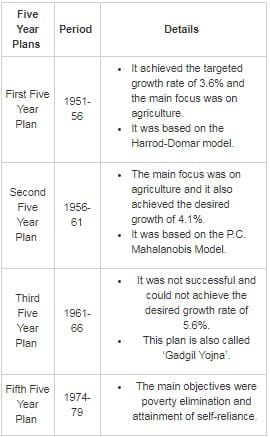RRB JE CBT 1 - Full Test 7 - Question 9

The “United Nations Environment Program” was established in pursuance of

Detailed Solution for RRB JE CBT 1 - Full Test 7 - Question 9

The United Nations Environment Programme has been established by the United Nations General Assembly in pursuance of the Stockholm Conference. The UNEP worked as catalyst stimulator and coordinator among the member states on the environmental action.

RRB JE CBT 1 - Full Test 7 - Question 10

Namdapha national park is situated in which of the following state?

Detailed Solution for RRB JE CBT 1 - Full Test 7 - Question 10

Namdapha National Park is the largest protected area in the Eastern Himalaya biodiversity hotspot and is located in Arunachal Pradesh in Northeast India.

RRB JE CBT 1 - Full Test 7 - Question 11

In which year 'Project Tiger' was launched?

Detailed Solution for RRB JE CBT 1 - Full Test 7 - Question 11

Project Tiger is a tiger conservation programme launched in 1973 by the Government of India during Prime Minister Indira Gandhi's tenure.

The project aims at ensuring

1) a viable population of Bengal Tigers in their natural habitats,

2) protecting them from extinction,

3) preserving areas of biological importance as a natural heritage

RRB JE CBT 1 - Full Test 7 - Question 12

Which musical instrument Ustad Bismillah Khan used to play?

Detailed Solution for RRB JE CBT 1 - Full Test 7 - Question 12
• Ustad Bismillah Khan used to play Shehnai.
• Ustad Bismillah Khan (1916 – 2006) (Real name is Qamaruddin Khan), was an Indian musician credited with popularizing the Shehnai.
• Shehnai is a musical instrument which is similar to the oboe (common in India, Pakistan, and Bangladesh.)
• He was honoured with India's highest civilian honour, the Bharat Ratna in 2001 thus becoming the third classical musician after M. S. Subbulakshmi and Ravi Shankar to be awarded this distinction.
• On his 102nd birth anniversary, he was honoured by Google with a Google doodle.
RRB JE CBT 1 - Full Test 7 - Question 13

“Lollapalooza Music festival” was celebrated in which of the following cities in August 2018?

Detailed Solution for RRB JE CBT 1 - Full Test 7 - Question 13
• “Lollapalooza Music festival” was held in August 2018 in Chicago.
• It started in the year 1991 and since then it is celebrated annually.
• It is a multi-genre (Alternative rock, punk rock, heavy metal, pop, hip hop, electronic ) delight which is celebrated every year in the USA.
• It includes music, dance, comedy and craft booths, etc.
RRB JE CBT 1 - Full Test 7 - Question 14

Who has received the Gandhi Peace Prize for 2018, on 16 January 2019?

Detailed Solution for RRB JE CBT 1 - Full Test 7 - Question 14
• The Gandhi Peace Prize for the years 2015,2016,2017 and 2018 were announced on 16 January 2019.
• Vivekananda Kendra got the prize for 2015.
• Akshaya Patra Foundation and Sulabh International got the prize for 2016.
• EkalAbhiyan Trust got the prize for 2017.
• Yohei Sasakawa got the prize for 2018.
• The annual prize was instituted by the Government of India in 1995.
RRB JE CBT 1 - Full Test 7 - Question 15

Who among the following is the author of the book titled 'Feisty At Fifty'?

Detailed Solution for RRB JE CBT 1 - Full Test 7 - Question 15
• The author of 'Feisty At Fifty' is Sudha Menon.
• 'Feisty at Fifty' is both, a moving personal story and the ultimate guide to make fifties, the most fabulous decade of your life.
• It is all about celebrating mid-life discoveries, and generally feeling great about ourselves.
RRB JE CBT 1 - Full Test 7 - Question 16

The colour of human skin is produced by _________.

Detailed Solution for RRB JE CBT 1 - Full Test 7 - Question 16
• The color of the skin is produced by the pigment Melanin. It is produced within the skin by cells called Melanocytes.
• Haemoglobin gives the red color to blood and helps in the transport of oxygen. Insulin controls the level of sugar in the blood.
RRB JE CBT 1 - Full Test 7 - Question 17

The study of skeleton is known as _________.

Detailed Solution for RRB JE CBT 1 - Full Test 7 - Question 17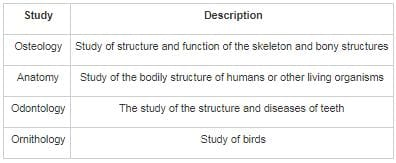RRB JE CBT 1 - Full Test 7 - Question 18

Which of the following cells secret insulin?

Detailed Solution for RRB JE CBT 1 - Full Test 7 - Question 18

Beta cells are unique cells in the pancreas that produce, store and release the hormone insulin. The main function of a beta cell is to produce and secrete insulin-the hormone which is responsible for regulating levels of glucose in the blood.

RRB JE CBT 1 - Full Test 7 - Question 19

Sleeping sickness is caused by _______

Detailed Solution for RRB JE CBT 1 - Full Test 7 - Question 19
• Sleeping sickness is caused by trypanosoma and that is why it is called African trypanosomiasis.
• Sleeping sickness is transmitted by tsetse fly.
• Sleeping sickness is sickness which happens in two stages.

Symptoms of the Sleeping Sickness:

1. First stage-Fever, headache and joint pain
2. Second stage- disturbance in sleeping pattern, personality changes and lethargy
RRB JE CBT 1 - Full Test 7 - Question 20

Which cell organelle is also known as “Suicidal Bag”?

Detailed Solution for RRB JE CBT 1 - Full Test 7 - Question 20
• Lysosomes are called “Suicidal Bags” because whenever a cell is damaged the lysosome bursts releasing all the digestive enzymes and thus killing the cell as well as itself.
• The lysosome is a membrane-bound organelle that contains an array of enzymes, capable of breaking down all types of biomolecules like proteins, carbohydrates and lipids.
• The lysosomes also act as the waste disposal system of the cell by digesting unwanted materials.
RRB JE CBT 1 - Full Test 7 - Question 21

What is Parthenocarpy?

Detailed Solution for RRB JE CBT 1 - Full Test 7 - Question 21

The development of fruits without fertilization of the ovules is called parthenocarpy. The fruits which are formed by this process are seedless. Example- banana, pineapple, etc.

RRB JE CBT 1 - Full Test 7 - Question 22

Which part of the coconut is edible?

Detailed Solution for RRB JE CBT 1 - Full Test 7 - Question 22

The edible part of coconut (Cocosnucifera) is endosperm.

RRB JE CBT 1 - Full Test 7 - Question 23

Leprosy is caused by

Detailed Solution for RRB JE CBT 1 - Full Test 7 - Question 23

Leprosy is a contagious disease that affects the skin, mucous membranes, and nerves, causing discoloration and lumps on the skin and, in severe cases, disfigurement and deformities. It is a long term infection caused by the bacteria Mycobacterium leprae andMycobacterium lepromatosis

RRB JE CBT 1 - Full Test 7 - Question 24

The Oropharynx is an organ is found in which part of the digestive system?

Detailed Solution for RRB JE CBT 1 - Full Test 7 - Question 24
• The pharynx is a part of the respiratory system and also a part of the digestive system.
• The pharynx has 3 parts: Nasopharynx, Oropharynx, and Laryngopharynx. The lower two part - the oropharynx and the laryngopharynx are involved in the digestive system. The Nasopharynx connects to the esophagus and it serves as a passageway for both air and food.
RRB JE CBT 1 - Full Test 7 - Question 25

An electric bulb is filled with

Detailed Solution for RRB JE CBT 1 - Full Test 7 - Question 25

Modern electric bulbs are usually filled with a mixture of argon and nitrogen. More rarely, some bulbs are filled with pure argon, krypton or xenon.

RRB JE CBT 1 - Full Test 7 - Question 26

Which one of the following obeys Ohm’s law?

Detailed Solution for RRB JE CBT 1 - Full Test 7 - Question 26
• Ohm’s law (V = IR) is applicable only to conductors and not to insulators. Even conductors like vacuum tubes, semiconductors, transistors, thermistors do not obey Ohm’s law.
• Vacuum tubes are non ohmic conductors. Even though it is known that ohm's law is followed by conductors. There are some conductors that do not follow ohm's law and they are known as non ohmic conductor.
RRB JE CBT 1 - Full Test 7 - Question 27

What is measured with the Nephelometer?

Detailed Solution for RRB JE CBT 1 - Full Test 7 - Question 27

Nephometer:

• An instrument designed to measure the amount of cloudiness
• It consists of a convex hemispherical mirror mapped into six parts.
• the amount of cloud coverage on the mirror is noted by the observer and then cloudiness is measured
RRB JE CBT 1 - Full Test 7 - Question 28

Which among the following is an example of an External Heat Engine?

Detailed Solution for RRB JE CBT 1 - Full Test 7 - Question 28
• Steam Engine is an example of an external heat engine.
• An external heat engine is such that the engine heat is produced outside the engine.
• In case of an internal heat engine, this heat is produced in the engine.
• Petrol and diesel engines are examples of internal heat engines.
RRB JE CBT 1 - Full Test 7 - Question 29

A real gas can act as an ideal gas at -

Detailed Solution for RRB JE CBT 1 - Full Test 7 - Question 29

At low pressure and high temperature, real gas can act as an ideal gas as the intermolecular force of attraction is negligible.

RRB JE CBT 1 - Full Test 7 - Question 30

Total internal reflection can take place only if

Detailed Solution for RRB JE CBT 1 - Full Test 7 - Question 30

In total internal reflection, the light must travel from a denser medium to rarer medium (e.g. glass to air or water to air).

RRB JE CBT 1 - Full Test 7 - Question 31

The oil in the wick of an oil Lamp rises up due to _________.

Detailed Solution for RRB JE CBT 1 - Full Test 7 - Question 31

Capillary action is the ability of a liquid to flow in narrow spaces without the assistance of, or even in opposition to, external forces like gravity. The liquid of the oil is drawn up into the wick by capillary action and vapourised by the heat of the flame.

RRB JE CBT 1 - Full Test 7 - Question 32

In a rechargeable cell what kind of energy is stored within the cell?

Detailed Solution for RRB JE CBT 1 - Full Test 7 - Question 32
• Chemical energy is stored within the rechargeable cells.
• Chemical energy is a type of potential energy stored in the bonds of chemical compounds and is released in the form of heat when the bonds between the atoms are broken.
• A rechargeable cell is a type of cell which can be charged, discharged into a load, and recharged many times.
• Electrical energy is converted into Chemical Energy when these cells are recharged.
RRB JE CBT 1 - Full Test 7 - Question 33

Plaster of Paris is obtained by heating ______.

Detailed Solution for RRB JE CBT 1 - Full Test 7 - Question 33
• Plaster of Paris is obtained by heating gypsum or calcium sulphate dihydrate to about 140-180 degree Celsius.
• When heated to such a temperature, gypsum formsPlaster of Paris.
• The name is derived from the large deposits of gypsum in the Montmartre hill in Paris.
RRB JE CBT 1 - Full Test 7 - Question 34

Bakelite is what type of polymer?

Detailed Solution for RRB JE CBT 1 - Full Test 7 - Question 34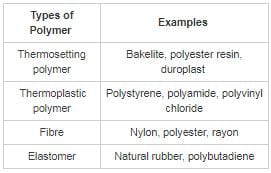RRB JE CBT 1 - Full Test 7 - Question 35

Which of the following elements is a p ­block element?

Detailed Solution for RRB JE CBT 1 - Full Test 7 - Question 35

Boron is a chemical element with symbol B and atomic number 5. It is a p-block element, produced entirely by cosmic ray spallation and supernovae and not by stellar nucleosynthesis. It is a low-abundance element in the Solar system and in the Earth's crust.

RRB JE CBT 1 - Full Test 7 - Question 36

The principal constituent of Pyrex glass is ________.

Detailed Solution for RRB JE CBT 1 - Full Test 7 - Question 36

Pyrex glass is a mixture of sodium and aluminium borosilicates. In ordinary glass, silica is the main constituent. In Pyrex glass some of the silica is replaced by boron oxide. Boron oxide expands very little when heated, thus, Pyrex glass does not crack on strong heating.

RRB JE CBT 1 - Full Test 7 - Question 37

Which device is used for the measurement of radioactivity?

Detailed Solution for RRB JE CBT 1 - Full Test 7 - Question 37

A Geiger counter (Geiger-Muller tube) is a device used for the detection and measurement of all types of radiation: alpha, beta and gamma radiation. Basically it consists of a pair of electrodes surrounded by a gas. The electrodes have a high voltage across them. The gas used is usually Helium or Argon.

RRB JE CBT 1 - Full Test 7 - Question 38

Bell metal contains Sn and ________.

Detailed Solution for RRB JE CBT 1 - Full Test 7 - Question 38
• Bell metal is a hard alloy used for making bells and different musical instruments.
• It is made of 80% copper and 20% tin (Sn) with some amount of Zinc and Lead.
• Bell metal is known for its resonance and attractive sound.
RRB JE CBT 1 - Full Test 7 - Question 39

Epsom Salt is an ore of which among the following metals?

Detailed Solution for RRB JE CBT 1 - Full Test 7 - Question 39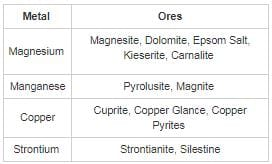RRB JE CBT 1 - Full Test 7 - Question 40

Van der Waal’s equation is related to the behavior of which of the following?

Detailed Solution for RRB JE CBT 1 - Full Test 7 - Question 40
• Van der Waal’s equation explains the behaviour of real gases with great accuracy and also the deviations of gas laws from ideal behaviour.
• The equation was proposed in the year 1873.
• It was a first step towards taking into account interaction forces which are acting between real gases molecules.
RRB JE CBT 1 - Full Test 7 - Question 41

Which among the following is cryogenic engines are used?

Detailed Solution for RRB JE CBT 1 - Full Test 7 - Question 41

Cryogenic fuels are used in the rocket technology as the extremely low temperature is essential for lifting and placing of heavier objects in space.

RRB JE CBT 1 - Full Test 7 - Question 42

What is the full form of GPRS?

Detailed Solution for RRB JE CBT 1 - Full Test 7 - Question 42

GPRS stands for General packet radio service.

GPRS is a packet oriented mobile data service on the 2G and 3G cellular communication system.

GPRS is used for transmitting data in the GSM network in from of packets.

RRB JE CBT 1 - Full Test 7 - Question 43

What is the unit of the physical quantity, Illuminance?

Detailed Solution for RRB JE CBT 1 - Full Test 7 - Question 43

The unit of illuminance is lux (lx). It measures luminous flux per unit area. It is equal to one lumen per square metre.

RRB JE CBT 1 - Full Test 7 - Question 44

Who gave the theory of Atom?

Detailed Solution for RRB JE CBT 1 - Full Test 7 - Question 44
• John Dalton, an English chemist, gave the theory of ‘Atom’.
• Dalton’s atomic theory states that all matter was composed of atoms.
• Atoms are the invisible and indestructible building blocks. All the atoms of the same elements are identical.
RRB JE CBT 1 - Full Test 7 - Question 45

Who is considered to be ‘the Father of modern genetics’?

Detailed Solution for RRB JE CBT 1 - Full Test 7 - Question 45

Gregor Mendel is usually considered to be the Father of modern genetics. Though farmers had known for centuries that crossbreeding of animals and plants could favor certain desirable traits, Mendel's pea plant experiments conducted between 1856 and 1863 established many of the rules of heredity.

RRB JE CBT 1 - Full Test 7 - Question 46

In the following question, by using which mathematical operators will the expression become correct?
17_21_8_13_52 = 36

Detailed Solution for RRB JE CBT 1 - Full Test 7 - Question 46

Using BODMAS rule,

1) +, ×, ÷ ,- ⇒ 17 + 21 × 8 ÷ 13 – 52

→ 17 + 12.92 – 52 = -22.08 ≠ 36

2) -, +, ×, ÷ ⇒ 17 – 21 + 8 × 13 ÷ 52

→ 17 – 21 + 2 = -2 ≠ 36

3) +, -, ×, ÷ ⇒ 17 + 21 – 8 × 13 ÷ 52

→ 17 + 21 – 2 = 36

4) ÷, ×, +, - ⇒ 17 ÷ 21 × 8 + 13 – 52

→ 6.47 + 13 – 52 = -32.53 ≠ 36

Hence, correct answer is “+, -, ×, ÷”.

RRB JE CBT 1 - Full Test 7 - Question 47

Direction: In the question below are given two statements and two conclusions. You have to take the given statements to be true even if they seem to be at variance from commonly known facts. Read all the conclusions and then decide which of the given conclusions logically follows from the given statements disregarding commonly known facts.

Statement I: All developer are girls.
Statement II: Some girls are CEO.

Conclusion I: No developer is CEO.
Conclusion II: Some developers are CEO.

Detailed Solution for RRB JE CBT 1 - Full Test 7 - Question 47

The least possible Venn diagram for the given statements is as follows,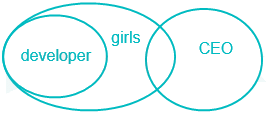Conclusion I: No developer is CEO → False

Conclusion II: Some developers are CEO → False

But conclusion I and II forms a complementary pair.

Hence either conclusion I or II follows.

RRB JE CBT 1 - Full Test 7 - Question 48

A word is represented by only one set of numbers as given in any one of the alternatives.

The sets of numbers given in the alternatives are represented by two classes of alphabets as in two matrices given below. The columns and rows of Matrix I are numbered – from 0 to 4 and that Matrix II are numbered from 5 to 9. A letter from these matrices can be represented first by its row and next by its column, e.g., ‘P’ can be represented by 00, 03 etc and N can be represented by 57, 65, etc. Identify the set for the word ‘CHAMP’.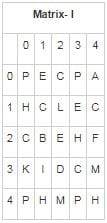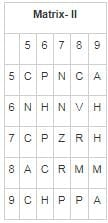Detailed Solution for RRB JE CBT 1 - Full Test 7 - Question 48

Let’s check each option,

1) 02, 44, 04, 96, 97 → CHAHP

2) 20, 66, 59, 42, 55 → CHAMC

3) 86, 79, 85, 89, 40 → CHAMP

4) 55, 66, 04, 34, 75 → CHAMC

Hence, the set which represent CHAMP is “86, 79, 85, 89, 40”.

RRB JE CBT 1 - Full Test 7 - Question 49

Direction: Arrange the given words in the order in which they will be arranged in a dictionary and choose the one that comes third.

I. Decide
II. Decimal
III. Decisive
IV. Decibel
V. Dedicate
VI. Decode

Detailed Solution for RRB JE CBT 1 - Full Test 7 - Question 49

On arranging given word as per dictionary order,

IV. Decibel
I. Decide
II. Decimal
III. Decisive
VI. Decode
V. Dedicate

Hence, “Decimal” comes third according to the dictionary.

RRB JE CBT 1 - Full Test 7 - Question 50

In the following question, find the odd word from the given alternatives.

Detailed Solution for RRB JE CBT 1 - Full Test 7 - Question 50

All of them are specialist in the field of medical, whereas Epistemologistis someone who studies how we know things.

Hence, “Epistemologist” is the odd one.

RRB JE CBT 1 - Full Test 7 - Question 51

Find the odd group of numbers from the given alternatives.

Detailed Solution for RRB JE CBT 1 - Full Test 7 - Question 51

1) 62 + 32 = 45; 452 = 2025

2) 52 + 42 = 41; 412 = 1681

3) 32 + 32 = 18; 182 = 324

4) 22 + 52 = 29; 292 = 841

Hence, “3, 3, 18, 330” is odd pair among the given option.

RRB JE CBT 1 - Full Test 7 - Question 52

In the following question find out the alternative which will replace the question mark.

HFBKD : KJHMH :: NNNOL : ?

Detailed Solution for RRB JE CBT 1 - Full Test 7 - Question 52

The logic here is as follows,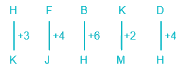Similarly,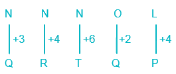Thus NNNOL is coded as QRTQP.

RRB JE CBT 1 - Full Test 7 - Question 53

Find the missing value denoted by ‘?’
82 : 216 :: ? : ?

Detailed Solution for RRB JE CBT 1 - Full Test 7 - Question 53

The logic here is as follows,

8 – 2 = 6; 63 = 216

1) 41 : 256,

4 – 1 = 3; 33 = 27

2) 63 : 27

6 – 3 = 3; 33 = 27

3) 52 : 64

5 – 2 = 3; 33 = 27

4) 93 : 676

9 – 3 = 6; 63 = 216

Hence, 63 : 27 is the correct pair.

RRB JE CBT 1 - Full Test 7 - Question 54

In the following question, find the related word from the given alternatives.
India : Rupee : : Denmark : ?

Detailed Solution for RRB JE CBT 1 - Full Test 7 - Question 54

Rupee is the currency of India. Similarly currency of Denmark is Krone.

Hence Denmark is related to Krone.

RRB JE CBT 1 - Full Test 7 - Question 55

If in a certain code language, CHROME is written as FKVSPH, then what would be the code for SWIGGY?

Detailed Solution for RRB JE CBT 1 - Full Test 7 - Question 55

The logic here is as follows,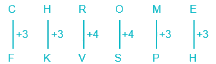Similarly,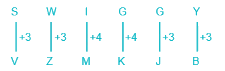Thus SWIGGY is coded as VZMKJB.

RRB JE CBT 1 - Full Test 7 - Question 56

Find the wrong term in the following series.

CWHK, FULL, ISPM, LQTN, OOYO

Detailed Solution for RRB JE CBT 1 - Full Test 7 - Question 56

The logic here is as follows,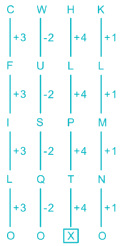So the wrong term in this series is OOYO.

RRB JE CBT 1 - Full Test 7 - Question 57

Which of the following answer figure patterns can be combined to make the question figure?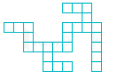Detailed Solution for RRB JE CBT 1 - Full Test 7 - Question 57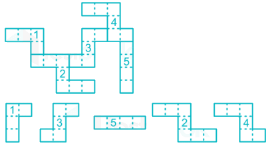Hence, the correct answer is option 3.

RRB JE CBT 1 - Full Test 7 - Question 58

Select the related figure from the given alternatives.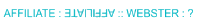Detailed Solution for RRB JE CBT 1 - Full Test 7 - Question 58

From first to the second figure, the image is water image of mirror image of the first figure.

Similarly,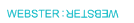Hence above image will be the answer.

RRB JE CBT 1 - Full Test 7 - Question 59

Which one set of letters, when placed at the gaps in the given letter series, shall complete it.

ABGGGDG, GABGGDG, ?, GGGABDG, GGGDABG

Detailed Solution for RRB JE CBT 1 - Full Test 7 - Question 59

Here first the group of AB moves 1 place in forward direction.

A and B will always remain beside each other.

Hence set ‘GGABGDG’ will complete the series.

RRB JE CBT 1 - Full Test 7 - Question 60

In making decisions about important questions, it is desirable to be able to distinguish between strong and weak arguments. Strong arguments are those, which are both important and directly related to the question. Weak arguments are those, which are of minor importance and also may not be directly related to the question or may be related to a trivial aspect of the question. The questions below are followed by two arguments each numbered I and II. You have to decide which of the two arguments is the strong argument and which is the weak argument.

Statement:
Should it be allowed to make movies with controversial plots?

Arguments:
I. Yes. A movie is made to entertain its audience and should have the freedom to have any plot.
II. No. Controversial plots might offend some groups of people.

Detailed Solution for RRB JE CBT 1 - Full Test 7 - Question 60

Most often than not, movies are a work of fiction and should be allowed to have any plot that would entertain the audience. Keeping a check on the plot of the movie would curb the right of creative freedom of its makers. People might end up getting offended but it is not the fault of the makers and not allowing them to freely make the movie is not a viable solution. Therefore, movies should be allowed to have any plot and option 1 is the right answer.

RRB JE CBT 1 - Full Test 7 - Question 61

Find the next term in the following series.
Define Problem, Measure, Analyze,?

Detailed Solution for RRB JE CBT 1 - Full Test 7 - Question 61

Here first you define the problem and the objectives, and then you measure it if any improvement is needed. Next step is to analyze the situation and the process follows to improve that, the next step is to improve the problem.

Thus the next term in the series is Improve.

RRB JE CBT 1 - Full Test 7 - Question 62

Directions: In the question given below, there is a statement followed by three assumptions numbered I, II and III. An assumption is something supposed or taken for granted. You have to consider the statement and assumptions and then decide which of the assumption(s) is/are implicit in the statement. You have to consider the statements to be true even if they seem to be at variance from commonly known facts.

Statement: Only a few months after fighting a drought, the Kerala government declared an imminent flood.

Assumptions:
I.
Kerala government declared a flood because they fought the drought.
II. Fighting a drought leads to floods.
III. Kerala is a disaster-prone region.

Detailed Solution for RRB JE CBT 1 - Full Test 7 - Question 62

Solution: The statement emphasizes on 2 potentially opposite forms of disasters, viz. drought and flood, Kerala is facing in a span of few months. So, we can assume that Kerala is prone to disasters. Hence assumption 3 is correct. The reason for the flood is not mentioned in the statement, so assumption 1 cannot be made. Similarly, no relation between the flood and the drought can be determined from the statement, so assumption 2 is also not true. Therefore, option 4 is the right answer.

RRB JE CBT 1 - Full Test 7 - Question 63

Study the following information carefully and answer the given questions.

In a certain code language,
“Agriculture is important” is coded as “@4 !3 *8”
“Nothing is improving” is coded as “*8 &1 %5”
“Agriculture field improving” is coded as “!3 &1 *3”

Which of the following means “nothing” in the coded language?

Detailed Solution for RRB JE CBT 1 - Full Test 7 - Question 63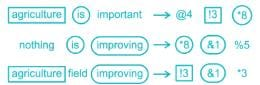Hence, “nothing” is coded as “%5”.

RRB JE CBT 1 - Full Test 7 - Question 64

Direction: In making decisions about important questions, it is desirable to be able to distinguish between strong and weak arguments. Strong arguments are those, which are both important and directly related to the question. Weak arguments are those, which are of minor importance and also may not be directly related to the question or may be related to a trivial aspect of the question. The questions below are followed by two arguments each numbered I and II. You have to decide which of the two arguments is the strong argument and which is the weak argument.

Statement:

Should there be a regular check on the income of the people by the Income Tax department?

Arguments:
I. Yes. A regular check by the Income Tax department would prevent tax evasions.
II. No. People would not agree to be regularly checked.

Detailed Solution for RRB JE CBT 1 - Full Test 7 - Question 64

Argument I provides us with a valid reason in favour of the regular checking. Regular checks by the Income Tax department would help keep a check on the black money generated in the economy and would prevent people from hiding their real income. Argument II makes an assumption that people would not like this regular checking. We cannot consider this assumption as it is not concrete. Therefore, option 1 is the correct answer.

RRB JE CBT 1 - Full Test 7 - Question 65

Study the information given below carefully and answer the question that follows.

Amit starts from his house towards the east direction, after walking a distance of 30 meters he turned left and walked 60 meters. He then turned right and walked a distance of 30 meters to reach the bank. From bank, he walked in north direction for 40 meters and then turned left. Finally, after walking for 60 meters he turned left and walked 20 meters to reach his final destination.

Q. What is the distance between the final position and his house and in which direction is the final destination with respect to the bank?

Detailed Solution for RRB JE CBT 1 - Full Test 7 - Question 65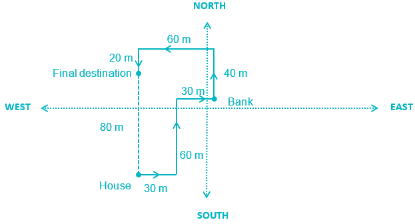The distance between final destination and his home is 80 meters and the final destination is in North West direction with respect to the Bank.

Hence, “80 meters, North West” is the correct answer.

RRB JE CBT 1 - Full Test 7 - Question 66

Select the possible fold of transparent paper sheet from the given options.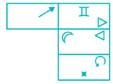Detailed Solution for RRB JE CBT 1 - Full Test 7 - Question 66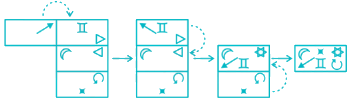Hence, the correct answer is option 2.

RRB JE CBT 1 - Full Test 7 - Question 67

There is a photograph of six people C, D, E, F, G, and H sitting in a row. Mahesh observes the following things while seeing the photograph. A number of people sitting to the left of G is equal to the number of people sitting to the right of H. F sits exactly between D and H such that either D or H sits at extreme ends. No person sits to the left of E. C is not the neighbor of E. Who was sitting second to the right of C while taking the photograph?

Detailed Solution for RRB JE CBT 1 - Full Test 7 - Question 67

According to the information given, from Mahesh's viewpoint,

1) No person sits to the left of E.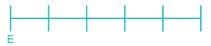2) F sits exactly between D and H such that either D or H sits at extreme ends.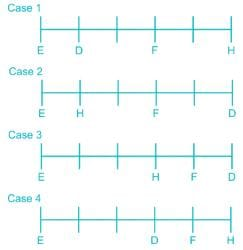3) Number of people sitting to the left of G is equal to the number of people sitting to the right of H.

Here case 1 and case 4 are eliminated as H is sitting at extreme right end but G cannot be placed at extreme left end as E is already sitting there.

4) C is not the neighbor of E.

Thus case 3 also gets eliminated.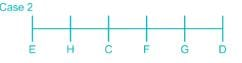As while taking photograph the left and right hands of the person are in opposite direction to that of the viewer of photograph, E was sitting second to the right of C while taking the photograph.

RRB JE CBT 1 - Full Test 7 - Question 68

Directions: In the question below are given a statement followed by three courses of action numbered I, II and III. A course of action is a step or administrative decision to be taken for improvement, follow-up or further action in regard to the problem, policy, etc. Consider the information given in the statement to be true, decide which of the suggested courses of action logically follow(s) for pursuing.

Statement: The air pollution index of the city, caused mainly due to vehicles, has reached a dangerously high level.

Courses of action:
I.
The vehicular traffic in the city should be controlled and improved in standards.
II. The public transport facility of the city should be improved.
III. Use of electric vehicles that do not emit any harmful gases should be promoted.

Detailed Solution for RRB JE CBT 1 - Full Test 7 - Question 68

Answer: 4. All of the above follow.

Solution: The air pollution index rises because of vehicles; hence controlling its number and improving its standard will be beneficial. So, action I follows. If there are better public transport systems available, people will use them instead of individual cars, which will reduce the overall traffic. Hence, action II follows. Promoting electric vehicles will also help in reducing the pollution index, therefore action III also follows. Hence, option 4 is the correct answer.

RRB JE CBT 1 - Full Test 7 - Question 69

In a family there are 10 persons A, B, C, P, Q, R, T, K, X, and G. There is the equal number of male and female members in the family. P is the father of A and B. Q is a mother in law of C who is the husband of B. T who is married to X is the mother of R, K, and C. C is the only son of X. G is the nephew of A. How is T related to G?

Detailed Solution for RRB JE CBT 1 - Full Test 7 - Question 69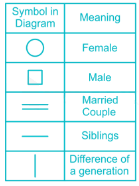According to the question,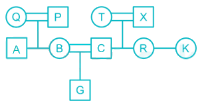Thus T is the grandmother of G.

RRB JE CBT 1 - Full Test 7 - Question 70

Which answer figure will complete the pattern in the following question figure?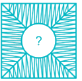Detailed Solution for RRB JE CBT 1 - Full Test 7 - Question 70Hence, figure 4 is the correct answer.

RRB JE CBT 1 - Full Test 7 - Question 71

Pipe A, B, and C can fill an empty tank alone in 6 hours, 8 hours and 12 hours respectively. A tank is initially 1/6 filled. Pipes A and B are simultaneously opened in the tank and closed after 2 hours. Now, if pipe C is opened in the tank, in how much time will it be filled?

Detailed Solution for RRB JE CBT 1 - Full Test 7 - Question 71

Part filled by A in 1 hr. = 1/6

Part filled by B in 1 hr. = 1/8

Part filled by C in 1 hr. = 1/12

Now, pipes A and B are opened for 2 hrs.

⇒ Part filled by A and B together in 2 hrs. = 2(1/6 + 1/8) = 2 × 7/24 = 7/12

∵ Tank is initially 1/6 filled,

Remaining part of tank to be filled = 1 - 1/6 - 7/12 = 1 - 3/4 = 1/4

∴ Pipe C will fill the remaining part in = (1/4)/(1/12) = 3 hrs

RRB JE CBT 1 - Full Test 7 - Question 72

If sin x + sin2x = 1, then value of cos2x + cos4 x is

Detailed Solution for RRB JE CBT 1 - Full Test 7 - Question 72

sin x + sinx = 1       ----(1)

cos2 x + cosx = ?        ----(2)

From equation (1)

sin x + sin2 x = 1 (sin2x + cos2x = 1)

sin x + 1 – cos2 x = 1

sin x = cosx

sinx = cosx

substituting value of cos2 x and cos4 x in (2)

cos2 x + cos4 x = sin x + sin2 x = 1

RRB JE CBT 1 - Full Test 7 - Question 73

When a student, weighing 38 kgs, left a class, the average weight of the remaining 69 students increased by 200 g. What is the average weight of the remaining 69 students?

Detailed Solution for RRB JE CBT 1 - Full Test 7 - Question 73

Let the average weight of the 69 students be A.

∴ total weight of the 69 of them will be 69A.

As question states, the total weight of the class = 69A + 38

When this student is also included, the average weight decreases by 0.2 kg;

⇒ (69A + 38)/70 = A - 0.2

⇒ 69A + 38 = 70A - 14

⇒ 70A - 69A = 38 + 14

⇒ A = 52

∴ Average weight of the remaining 69 students = 52 kg

RRB JE CBT 1 - Full Test 7 - Question 74

If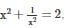then what is the value of x6?

Detailed Solution for RRB JE CBT 1 - Full Test 7 - Question 74

x2 + 1 / x2 = 2

⇒ x2 + 1 / x2 - 2 = 0

⇒ (x - 1 / x)2 = 0

⇒ x - 1 / x = 0

⇒ x = 1 / x

⇒ x= 1

∴ x = 1, -1

Hence, x6 = 1

RRB JE CBT 1 - Full Test 7 - Question 75

If 3, 5, 7,… is an A.P., determine the common ratio if its 5th, 16th, 49th term are in G.P.

Detailed Solution for RRB JE CBT 1 - Full Test 7 - Question 75

For the given A.P.,

First term, a = 3 and common difference, d = 2

The nth term = a + (n – 1) d

5th term = 3 + 4 × 2 = 11

16th term = 3 + 15 × 2 = 33

49th term = 3 + 48 × 2 = 99

Hence, the G.P. is 11, 33, 99

Since, G.P. is of the form a, ar, ar2

Hence the common ratio, r = 33/11 = 3

RRB JE CBT 1 - Full Test 7 - Question 76

If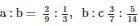and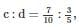then a : b : c : d is

Detailed Solution for RRB JE CBT 1 - Full Test 7 - Question 76

a : b : c : d

2/9 : 1/3 : 1/3 : 1/3

3/7 : 3/7 : 5/14 : 5/14

7/10 : 7/10 : 7/10 : 3/5

42/630 : 21/210 : 35/420 : 15/210

⇒ 42/3 : 21/1 : 35/2 : 15/1

⇒ 28 : 42 : 35 : 30

RRB JE CBT 1 - Full Test 7 - Question 77

Find the roots of the equation if the roots of the quadratic equation 7m2 – 14m + a = 0 are reciprocal to each other.

Detailed Solution for RRB JE CBT 1 - Full Test 7 - Question 77

We know if ax2 + bx + c = 0 is a quadratic equation, product of the roots = (c/a)

⇒ In the given problem a = 7, b = -14, c = a

If the roots of the equation are reciprocal to each other, then the product of the roots = 1

⇒ (a/7) = 1

⇒ a = 7

On putting ‘a’ = 7 in the original equation, the equation will now be 7m2 – 14m + 7 = 0

⇒ m2 – 2m + 1 = 0 (Dividing the previous equation with 7 on both sides)

⇒ m2 – m – m + 1 = 0

⇒ m (m – 1) – (m -1) = 0

⇒ (m - 1) (m – 1) = 0

⇒ (m - 1)2 = 0

⇒ m = 1

∴ So, the roots of the quadratic equation are 1, 1

RRB JE CBT 1 - Full Test 7 - Question 78

Out of the five subjects, the average marks of Suman in first 3 Subjects was 81 and the average marks in the last 3 subjects were 74. If her marks in the third subject were 65, then what were her average marks in all the subjects?

Detailed Solution for RRB JE CBT 1 - Full Test 7 - Question 78

Let the five subjects are a, b, c, d and e

Marks in third subject c = 65 given

Average marks in first three subjects = 81

⇒ (a + b + c)/3 = 81

⇒ a + b + c = 243

Average marks in last three subjects = 74

⇒ (c + d + e)/3 = 74

⇒ c + d + e = 222

⇒ 65 + d + e = 222

⇒ d + e = 222 – 65 = 157

Average marks of suman in all sub. = (a + b + c + d + e)/5 = (243 + 157)/5

∴ Average marks = 400/5 = 80

RRB JE CBT 1 - Full Test 7 - Question 79

How many zeroes are there in the product 48 × 3512?

Detailed Solution for RRB JE CBT 1 - Full Test 7 - Question 79

5 × 2 = 10

⇒ The number of 2’s and 5’s in the product determines the number of zeroes in it.

48 × 3512

= (22)× (7 × 5)12

= 216 × 712 × 512 (∵ (am)n = amn (a × b)m = a× bm)

=712 × 2× (2 × 5)12

∴ Number of zeroes in the product 48 × 3512 are 12.

RRB JE CBT 1 - Full Test 7 - Question 80

Two persons are standing on two sides of a pole. The angle of elevation of the top of the pole from A is 300 and from B is 450. If the height of the pole is 100 cm, find the distance between two persons.

Detailed Solution for RRB JE CBT 1 - Full Test 7 - Question 80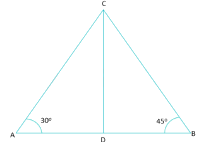Let CD be the pole and A and B be positions of two persons

⇒ Then CD = 100 cm and ∠DAC = 300 and ∠DBC = 450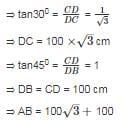⇒ AB = 273 cm

∴ The distance between two persons = 273 cm

RRB JE CBT 1 - Full Test 7 - Question 81

A sum of Rs 3100 is lent out at simple interest in two parts. One at 8% per annum and another at 6% per annum. If the total annual interest is Rs 212, then what is the money (in Rs) lent at rate of 8%?

Detailed Solution for RRB JE CBT 1 - Full Test 7 - Question 81

Let P= x and P= 3100 - x

Simple Interest =(P × R × T)/100

Where P is Principle amount, R is rate of interest, T is time in years.

Total annual interest = I+ I= (8 × x × 1)/100 + [(3100 - x) × 6 × 1]/100

x = Rs. 1300

RRB JE CBT 1 - Full Test 7 - Question 82

A boat cover row 12 km upstream in 4 hours and can cover the same distance downstream in 3 hours. What is the speed (in km/hr) of the boat in still water?

Detailed Solution for RRB JE CBT 1 - Full Test 7 - Question 82

Let the speed of boat = x km/hr

& the speed of stream = y km/hr

According to the question

⇒ 12/(x - y) = 4

⇒ x - y = 3      ----(1)

⇒ 12/(x + y) = 3

⇒ x + y = 4     ----(2)

From equation (1) and equation (2)

⇒ x = 3.5 and y = 0.5

So, speed of boat in still water is 3.5 km/h

RRB JE CBT 1 - Full Test 7 - Question 83

Directions: The following graph shows the registration of new vehicles (in thousands) in a State during July - December 2010. Study the graph and answer questions.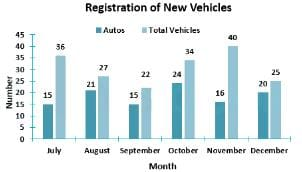Q. In which month is the percentage growth in registration of total vehicles maximum?

Detailed Solution for RRB JE CBT 1 - Full Test 7 - Question 83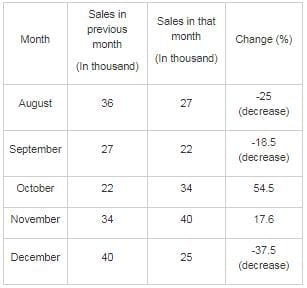RRB JE CBT 1 - Full Test 7 - Question 84

In an examination, a student scores 4 marks for every correct answer and loses 1 mark for every wrong answer. If he attempts all 75 questions and secures 125 marks, the number of questions he attempts correctly is

Detailed Solution for RRB JE CBT 1 - Full Test 7 - Question 84

Let the number of questions attempted correctly be X and those attempted wrong be Y.

Given,

Total question attempted= 75

⇒ X + Y = 75       …………………(1)

Also total score is 125. Since 4 marks is awarded for every correct answer and 1 marks is lost for every wrong answer. Thus

⇒(X × 4) +(Y × (-1)) = 125

⇒ 4X – Y = 125       ………………….(2)

Adding (1) and (2) both sides, we get

⇒ 5X = 200

⇒ X = 40

Hence total number of questions attempted correctly is 40.

RRB JE CBT 1 - Full Test 7 - Question 85

Vessel A contains milk and water in the ratio 5 ∶ 1. Vessel B contains milk and water in the proportion 4 ∶ 5.In what proportion should quantities be taken from B & A to form a mixture in which milk and water are in the ratio 5 ∶ 4?

Detailed Solution for RRB JE CBT 1 - Full Test 7 - Question 85

In Vessel B, the proportion of milk in 4/((4 + 5) ) = 4/9.

In vessel A, the proportion of milk is 5/(5 + 1) = 5/6.

The amount of milk in the mixture = 5/((5 + 4)) = 5/9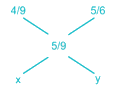X = (5/6) - (5/9) = 5/18

Y = (5/9) - (4/9) = 1/9

The ratio is (5/18) ∶ (1/9) = 5 ∶ 2

RRB JE CBT 1 - Full Test 7 - Question 86

The factors of x16 + x8 + 1 are

Detailed Solution for RRB JE CBT 1 - Full Test 7 - Question 86

Formula:

a2 – b2 = (a + b)(a – b)

Given,

x16 + x8 + 1

= x16 + 2x8 + 1 – x8

= (x8 + 1)2 – (x4)2

= (x8 + 1 – x4)(x8 + 1 + x4)

= (x8 + 1 – x4)((x4 + 1)2 – (x2)2)

= (x8 + 1 – x4)(x+ 1 – x2)(x4 + 1 + x2)

= (x8 + 1 – x4)(x+ 1 – x2)((x2 + 1)2 - x2)

= (x+ 1 – x4),(x4 + 1 - x2)(x2 + 1 + x)(x2 + 1 - x)

Thus the factors are:

(x+ 1 – x4), (x4 + 1 - x2) , (x2 + 1 + x), (x2 + 1 - x)

RRB JE CBT 1 - Full Test 7 - Question 87

Two trains, whose ratio of speed is 5 ∶ 7, can cross a vertical pole in 12 seconds and 15 seconds respectively. Find the time in which they will cross each other if they are going in opposite directions.

Detailed Solution for RRB JE CBT 1 - Full Test 7 - Question 87

Suppose the speeds of train are 5x and 7x km/hr;

∴ Length of 1st train = 60x

Length of 2nd train = 105x

∴ Time in which they will cross each other = (60x + 105x) / (5x + 7x) = 165/12 = 13.75 seconds

RRB JE CBT 1 - Full Test 7 - Question 88

The price of 1 Jeans increased by 20% and that of 1 watch decreased by 10%. If the initial price of 20 jeans is equal to that of 2 watches, then the new price of 45 jeans is equal to that of:

Detailed Solution for RRB JE CBT 1 - Full Test 7 - Question 88

Let the initial price of a jeans and a watch be Rs. ‘x’ and Rs. ‘y’ respectively

Hence, we have, 20x = 2y

⇒ x = y/10      ----(1)

New price of a jeans = (100 + 20)% of x = 1.2x

New price of 45 jeans = 45 × 1.2x = 54x

Substituting from (1),

New price of 45 jeans = 54 × (y/10) = 5.4y

New price of a watch = (100 – 10)% of y = 0.9y

No. of watches costing equal to 45 jeans = 5.4y/0.9y = 6

∴ The new price of 45 jeans is equal to that of 6 watches

RRB JE CBT 1 - Full Test 7 - Question 89

If 15 men take 21 days of 8 hours each to do a piece of work, then what is the number of days of 6 hours each that 21 women would take if 3 women would as much work as 2 men?

Detailed Solution for RRB JE CBT 1 - Full Test 7 - Question 89

Since 3 women would as much work as 2 men;

Ratio of efficiencies of Man and Woman = 3 ∶ 2 Or 2M = 3W

15 men take 21 days of 8 hours each to do a piece of work, let suppose 21 women take x days of 6 hours each to do that work;

∴ (15M × 21 × 8) = (21W × 6 × x)

Putting 2M = 3W;

⇒ (15M × 21 × 8) = (14M × 6 × x)

⇒ x = 30

∴ 21 women will take 30 days of 6 hours each to do the work.

RRB JE CBT 1 - Full Test 7 - Question 90

How many kg of rice at Rs. 54 per kg must be mixed with 30 kg of rice at Rs. 40 per kg, so that on selling the mixture at Rs 48.4 per kg, there will be a profit of 10%?

Detailed Solution for RRB JE CBT 1 - Full Test 7 - Question 90

Let ‘x’ kg of rice at Rs. 54 per kg be mixed

Total weight of mixture = (x + 30) kg

Cost price of (x + 30) kg mixture = 54x + 30 × 40 = 54x + 1200

⇒ Cost price of 1 kg mixture = (54x + 1200)/(x + 30)

Now, when selling price is Rs. 48.4 per kg, profit is 10%

⇒ Cost price of 1 kg mixture + 10% of Cost price = Selling price

⇒ 110% of Cost price of 1 kg mixture = Selling price

⇒ Cost price of 1 kg mixture = 48.4/1.1 = Rs. 44

Hence, we get,

⇒ (54x + 1200)/(x + 30) = 44

⇒ 54x + 1200 = 44x + 1320

⇒ 10x = 120

⇒ x = 120/10 = 12

∴ 12 kg of rice must be added

RRB JE CBT 1 - Full Test 7 - Question 91

To travel 660 km, an Express train takes 10 hours more than Rajdhani. If however, the speed of the Express train is doubled, it takes 7 hours less than Rajdhani. The speed of Rajdhani is

Detailed Solution for RRB JE CBT 1 - Full Test 7 - Question 91

Let time taken by Rajdhani be y hrs

Let the speed of Express train be x km/hr

Time taken by Express train = 660/x

660/x = y + 10      ----(1)

New speed of Express train be 2x km/hr

Time taken by Express train = 2x/660

660/2x = y - 7      ----(2)

From (1) and (2) we get x = (660/34) km/hr and y = 24 hr

Speed of Rajdhani = 660/24 = 27.5 km/hr

RRB JE CBT 1 - Full Test 7 - Question 92

What is the value of [(cos32θ + 3cos2θ) ÷ (cos6θ - sin6θ)]?

Detailed Solution for RRB JE CBT 1 - Full Test 7 - Question 92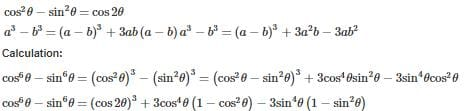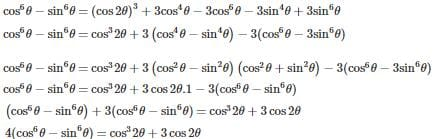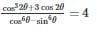Alternate:
Put θ = 0,

⇒ [(cos32θ + 3cos2θ) ÷ (cos6θ - sin6θ)] = [(cos3(0) + 3cos(0)] ÷ (cos6(0) - sin6(0)]

⇒ [(1 + 3) ÷ (1 - 0)] = 4

∴ [(cos32θ + 3cos2θ) ÷ (cos6θ - sin6θ)] = 4

RRB JE CBT 1 - Full Test 7 - Question 93

If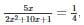then the value of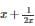is

Detailed Solution for RRB JE CBT 1 - Full Test 7 - Question 93

Given,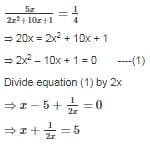RRB JE CBT 1 - Full Test 7 - Question 94

Mukesh sells two shirts. The cost price of the first shirt is equal to the selling price of the second shirt. The first shirt is sold at a profit of 30% and the second shirt is sold at a loss of 30%. What is the ratio of the selling price of the first shirt to the cost price of the second shirt?

Detailed Solution for RRB JE CBT 1 - Full Test 7 - Question 94

First shirt is sold at a profit of 30%

We write 30% = 3/10

Let CP of the first shirt be 10 and profit be 3

So, SP of the first shirt = 10 + 3 = 13

(CP)1/(SP)1 = 10/13      ----(1)

Similarly second shirt is sold at a loss of 30%

We write 30% = 3/10

Let CP of the second shirt be 10 and loss be 3

So, SP of the second shirt be = 10 – 3 = 7

(CP)2/(SP)2 = 10/7      ----(2)

Given, CP of the first shirt is equal to the SP of the second shirt.

Multiply by 7 in equation (1) and multiply by 10 in equation (2) we get

CP)1/(SP)1 = 70/91

(CP)2/(SP)2 = 100/70

∴ ratio of the selling price of the first shirt to the cost price of the second shirt is 91 : 100

RRB JE CBT 1 - Full Test 7 - Question 95

A conical vessel of base radius 16 cm and height 12 cm is filled with water. If the water leaks through a hole at the bottom of the conical vessel into a cylindrical jar of radius 8 cm, then find the level of the water in the jar after the water has leaked completely.

Detailed Solution for RRB JE CBT 1 - Full Test 7 - Question 95

⇒ given: Radius of cone, r = 16 cm, Height, h = 12 cm

⇒ Radius of cylindrical jar, r1 = 8 cm, height, h1 = x

⇒ Volume remains the same in this case

⇒ Volume of cone = Volume of Cylinder

⇒ (1/3) × (π × r2 × h) = (π × r12 × h1)

⇒ (1/3) × (π × 162 × 12) = (π × 82 × x)

⇒ 16 × 16 × 4 = 8 × 8 × x

∴ x = 16 cm

RRB JE CBT 1 - Full Test 7 - Question 96

Rs. 3000 are divided amongst A, B and C, so that if Rs. 20, Rs. 40 and Rs. 60 be taken from their shares respectively, they will have money in the ratio 3 ∶ 4 ∶ 5. Find the shares of C.

Detailed Solution for RRB JE CBT 1 - Full Test 7 - Question 96

Total decrease = 3000 - 120 = 2880

C’s share = 5/12 × 2880 = 5 × 240 = 1200

Share of C out of 3000 = 1200 + 60 = 1260

RRB JE CBT 1 - Full Test 7 - Question 97

2 men and 3 women undertook a piece of work for Rs. 12,000. 2 men can do the work in 12 days and 3 women can do the same work in 15 days. With the assistance of 5 children, they finished the work in 5 days. What is the share of 1 child?

Detailed Solution for RRB JE CBT 1 - Full Test 7 - Question 97

Work done by 2 men in 1 day = 1/12

Work done by 3 women in 1 day = 1/15

Work done by (2 men + 3 women + 5 children) in 1 day = 1/5

∴ Work done by 5 children in 1 day = 1/5 – 1/12 – 1/15 = 1/20

⇒ 5 children alone can do the work in 20 days

Ratio of their time (2 Men ∶ 3 Women ∶ 5 Children) = 12 ∶ 15 ∶ 20

We know, efficiency is inversely proportional to the time

∴ Ratio of their efficiency (2 Men ∶ 3 Women ∶ 5 Children) = 1/12 ∶ 1/15 ∶ 1/20 = 5 ∶ 4 ∶ 3

So, 5 + 4 + 3 = 12 unit = 12000

⇒ 3 unit = 3000

⇒ Share of 5 children is 3000

∴ Share of 1 child = 3000/5 = 600

RRB JE CBT 1 - Full Test 7 - Question 98

A same principal is invested in schemes of compound interest and simple interest. The interest obtained in compound interest and simple interest schemes for two years are Rs. 630 and Rs. 600 respectively. If the rate of interest is 10%, then what is the total principal (in Rs.)?

Detailed Solution for RRB JE CBT 1 - Full Test 7 - Question 98

Simple interest = (P × r × t)/100

P = Principal

t = Time period

r = Rate of interest

Given,

t = 2 years

r = 10%

Simple Interest = Rs. 600

600 = (P × 2 × 10)/100

⇒ P = 3000

Compound Interest = [P(1 + r/100)t - 1]

Where,

CI = Compound interest

P = Principal

r = Rate of interest

t = Time period

Given,

t = 2 years

r = 10%

Compound Interest = Rs. 660

630 = [P(1 + 10/100)2 - 1]

630 = 21P/100

P = 3000

Previous

RRB JE CBT 1 - Full Test 7 - Question 99

Every month Rahul saves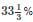of his income. After saving he spends 3/4th of the amount on home & food and the rest of money on transportation. If the amount saved every month is Rs. 12000 then, find the amount he spends on transportation?

Detailed Solution for RRB JE CBT 1 - Full Test 7 - Question 99

Expenditure = Total salary - Savings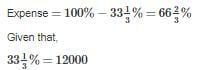So, 100 % salary will be = [12000 × 100]/ [100/3] = 36,000

So, his monthly expenses are = 36000 – 12000 = Rs. 24,000

According to the question,

Expense for Home & Food = 3/4 × 24000 = Rs. 18000

So, expense on transport = 24000 – 18000 = Rs. 6000

∴ So Rahul spend Rs. 6000 in transportation

RRB JE CBT 1 - Full Test 7 - Question 100

What number should be subtracted from each of the numbers 54, 71, 75 and 99, so that the reminders may be proportional?

Detailed Solution for RRB JE CBT 1 - Full Test 7 - Question 100

If x be the number, then 54 - x, 71 - x, 75 - x and 99 - x are in proportion.

(54 - x) (99 - x) = (71 - x) (75 - x)

5346 - 153x + x2 = x2 - 146x + 5325

X = 3

## RRB JE (Railways Junior Engineer) CBT Mock Test Series

19 tests
Information about RRB JE CBT 1 - Full Test 7 Page
In this test you can find the Exam questions for RRB JE CBT 1 - Full Test 7 solved & explained in the simplest way possible. Besides giving Questions and answers for RRB JE CBT 1 - Full Test 7, EduRev gives you an ample number of Online tests for practice

## RRB JE (Railways Junior Engineer) CBT Mock Test Series

19 tests(Scan QR code)